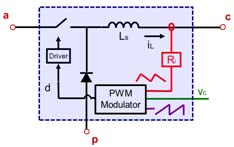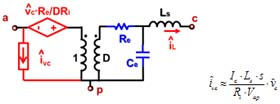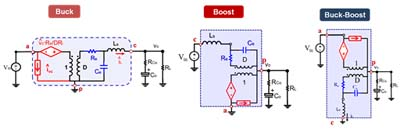LIBRARY

# Unified Three-terminal Switch Model for Current Mode Controls

Year: 2010Fig. 1. Common Structure of Current Mode Control Converter.
Current mode controls with various implementations, including constant frequency peak-current mode control, valley-current mode control, charge control as well as variable frequency constant on-time control and constant off-time control, have been widely used in the power converters. Discrete time and sample-data model is only valid for constant frequency modulations. To model variable frequency control, describing function method based on continuous time domain analysis was introduced by Jian Li. However, the analysis is based on given topology and the mathematical process is too complicated to be extended to model for hundreds of other topologies.

CPES proposed a unified three-terminal switch model for current mode controls. By identifying the invariant structure in current mode control PWM converter, which consists of active switch, passive switch and closed current loop, the terminal current and voltage relationships are studied and represented by equivalent circuit. Small signal model for current mode control PWM converter, which is good beyond half of switching frequency for forementioned control schemes, can be obtained by simple cyclic permutation and substitution of the PWM switch with its equivalent circuit.Fig. 2. Three-terminal Switch for Current Mode Control.Fig. 3. Applications in familiar converters.